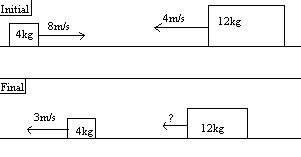# Simple momentum problem

## Homework Statement

A small block m=4kg and a large block m=12kg have a head on collision on a frictionless table. Before the collision, the small block is traveling 8m/s to the right and the large block is traveling 4m/s to the left.A. If the small block bounces back at 3m/s to the left, find the final velocity of the large block.

B. Suppose instead the two blocks stick together after they collide. How many Joules of energy would be lost?

## Homework Equations

$$p=mv$$

$$KE=\frac{1}{2}mv^{2}$$

## The Attempt at a Solution

I will define to the right to be the positive x direction.
First I will calculate initial momentum for both blocks.

$$p_{i a}=mv$$
$$p_{i a}=(4kg)(8m/s)$$
$$p_{i a}=32kg m/s$$

$$p_{i b}=mv$$
$$p_{i b}=(12kg)(-4m/s)$$
$$p_{i b}=-48kg m/s$$

$$p_{i}=-16kg m/s$$

Now final momentum:

$$p_{f a}=mv$$
$$p_{f a}=(4kg)(-3m/s)$$
$$p_{f a}=-12kg m/s$$

$$p_{f b}=mv$$
$$p_{f b}=(12kg)v$$
$$p_{f b}=12v$$

$$p_{f}=-12kg m/s + 12v$$

If momentum is conserved, then

$$p_{i}=p_{f}$$

$$-16kg m/s=-12kg m/s + 12v$$

$$v=\frac{-1}{3}$$

kuruman
Homework Helper
Gold Member
So far you are correct. Is there a question you wish to ask?

Oh, great. I had a feeling I was on the wrong track.

For part B, I need to find the energy. It is all Kinetic.

$$KE_{i}=\frac{1}{2}mv_{a}^{2}+\frac{1}{2}mv_{b}^{2}$$

$$KE_{i}=\frac{1}{2}(4)(8)^{2}+\frac{1}{2}(12)(4)^{2}$$

$$KE_{i}=128+96$$

$$KE_{i}=224J$$

Now the final kinetic energy is where I am getting confused.

$$KE_{f}=\frac{1}{2}mv_{a}^{2}+\frac{1}{2}mv_{b}^{2}$$

How can I find the velocity of the two blocks?

Actually, for part B I did it another way:

$$p_{i}=32+-48$$

$$p_{i}=-16$$

$$p_{f}=16v$$

$$p_{i}=p_{f}$$

$$-16=16v$$

$$v=-1m/s, or 1 m/s to the left$$

$$KE_{i}=\frac{1}{2}mv^{2}+\frac{1}{2}mv^{2}$$

$$KE_{i}=\frac{1}{2}(4)(8)^{2}+\frac{1}{2}(12)(4)^{2}$$

$$KE_{i}=224J$$

$$KE_{f}=\frac{1}{2}mv^{2}$$

$$KE_{f}=\frac{1}{2}(16)(1)^{2}$$

$$KE_{f}=8J$$

$$KE_{i}-KE_{f}=E_{lost}$$

$$KE_{i}-KE_{f}=E_{lost}$$

$$224-8=E_{lost}$$

$$E_{lost}=216J$$

kuruman